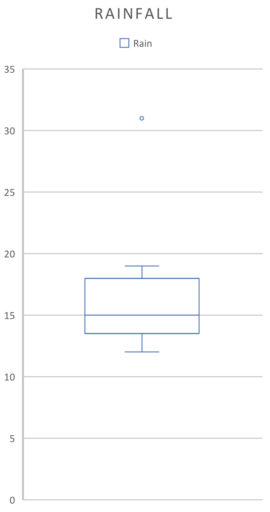In this post, we’ll define the mean, median, and mode. Along the way, we’ll work through an example of how to find each of them. We’ll also talk about why the median is responds less to outliers than the mean does, a fact which can sometimes be crucial in solving a GRE problem.

Finding the Mean

The mean (or average) of a variable is found by adding up all of the values of that variable and then dividing by the number of observations. So to go back to our lemonade example:Finding the Median

Now, finding the median is a little trickier. Imagine lining up all of the values for your variable from least to greatest. Then, the median is the one in the middle. So for example, if we have the data:We order it from least to greatest to get:And then we pick the middle value, namely.

Sometimes, if there is a long string of values, it will be hard to see which value is the middle one. So take the data:We order it from least to greatest:And then we simply cross off the numbers at the end, both the left-most and right-most observation, to get:

1, 1, 1, 1, 2, 2, 3, 3, 3, 3, 4, 6, 7, 8, 9

And we repeat:

1, 1, 1, 1, 2, 2, 3, 3, 3, 3, 4, 6, 7, 8, 9

Until we get:

1, 1, 1, 1, 2, 2, 3, 3, 3, 3, 4, 6, 7, 8, 9

And thus the median is 3.

Sometimes we will have an even number of observations. So suppose we have the data:Crossing through the numbers on the end, we get:

1, 2, 3, 4

But now, it seems, we are stuck. For the median is a single number - not two numbers! And yet if we cross out any more, we will eliminate all of our numbers.

In these cases, we say that the median is the average of the middle two numbers. Thus, the median in the above case isSo in summary, the median is either the middle number or, if you have two middle numbers, the average of those two middle numbers. Now, we try to find the median for our lemonade example:Finding the Mode

Finally, the mode is the value that occurs most often. So, if you have the data:Then the mode is.

Now, a set of numbers can have more than one mode, as in this example:Here, since bothandoccur three times (and no other number occurs three or more times), they are both modes.

Often, to find the mode it can help to put the numbers in ascending order (so you can see how often certain values are repeated). To return to our lemonade example:Median is More Resilient to Outliers

Finally, one property of the median is that it is "more resilient to outliers." What does this mean? First, let's be clear on what an outlier is: an outlier is a data value that is very far from many of the other observations. So suppose you have some data on how many days in a month it rains:But one month, you have constant downpours and so you getas a new observation. Your new data is:But theis quite far from all of the other observations. If we were to graph this data on a boxplot:We would see thatsticks out like a sore thumb. That's how you know it is an outlier. (There are more precise definitions of an outlier, but you won't need them for the GRE).

Now, when we say that the median is "more resilient" to outliers than the mean, we are saying that if we add an outlier to our data, the median is affected less than the mean. In other words, the change to the median will be less than the change to the mean.

Let's confirm that this is the case in our above example. To do so, we will compute the old mean/median, the new mean/median and compare them.

The old mean is:The old median is:since both 14 and 15 are in the middle ofThe new mean is:.
The new median is:.

So we can see that the new mean ishigher than the old mean, whereas the median only increased by. Thus, the median changed less than the mean did, exactly as predicted.

Why is the Median More Resilient?

Now, you might wonder why the median is more resilient than the mean to outliers. You won't need to know this for the GRE, but it might help in remembering which one is more resilient.

Here's why. When you add a new value to the data set, the median doesn't really care how large/small the new number is. All that matters is whether it is larger than the previous median or smaller. If it is larger than the previous median, then the new median moves a number to the right. If it is smaller, the new median moves a number to the left. But the mean does care about how large/small the new number is. So if the new number is an outlier, then it is, by definition, really large or really small compared to the other numbers. So the mean responds a lot to this new number whereas the median just moves a number to the right/left. That's why outliers affect the mean more than they do the median.

Practice Problems

1. Suppose we have the following heights of a kindergarten class (in inches): 29, 31, 33, 33, 37, 28, 29, 30. Find the mean, median, and mode of this data.
2. Suppose the ruler we used to measure everyone's height was mislabeled: in fact, everyone is five inches taller than the ruler suggests. Take the data in question 1, adjust for this fact, and find the new mean, median, and mode.
3. A new kid who is remarkably tall (60 inches) joins the class. Which statistic is more affected by this change, the median or the mean?